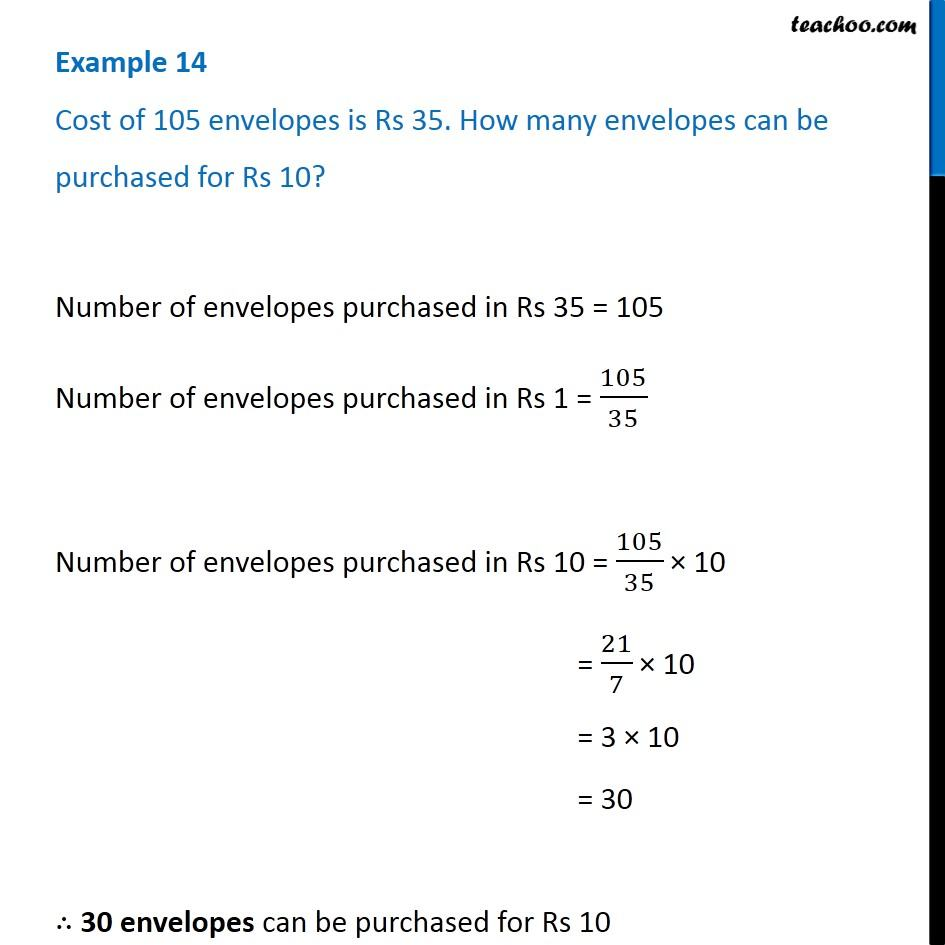Subscribe to our Youtube Channel - https://you.tube/teachoo

1. Chapter 12 Class 6 Ratio And Proportion
2. Concept wise
3. Unitary Method

Transcript

Example 14 Cost of 1Number of envelopes purchased in Rs 35 = 105 Number of envelopes purchased in Rs 1 = 105/35 Number of envelopes purchased in Rs 10 = 105/35 × 10 = 21/7 × 10 = 3 × 10 = 30 ∴ 30 envelopes can be purchased for Rs 10 05 envelopes is Rs 35. How many envelopes can be purchased for Rs 10?

Unitary Method